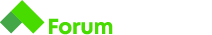# Проблем със задача 7 Interest C#

Здравейте, имам проблем със закръгляването на резултата до втората цифра.

Условие на задачата:

# Interest

You have deposited a sum into your bank account for 3 years. The bank has announced an interest of 5% per year. Each time the interest is calculated and added to your deposit. You have to calculate the amount in your deposit for each year.

## Input

• On the only line you will receive a number (n) with the sum deposited

## Output

• You should print the amount in your deposit for each of the 3 years

## Constraints

• You must print the number with two numbers after the decimal point. The rules of math for rounding apply here

## Input

``````1000
``````

## Output

``````1050.00
1102.50
1157.63
``````

## Input

``````2000
``````

## Output

``````2100.00
2205.00
2315.25
``````

ето ти примери как да форматираш double или float:

``````String.Format("{0:0.00}", 123.4567);      // "123.46"
String.Format("{0:0.00}", 123.4);         // "123.40"
String.Format("{0:0.00}", 123.0);         // "123.00"
``````

би трябвало да ти свършат работа

Благодаря за примерите, но не ми закръгля както се очаква в задачата.
Console.WriteLine(Math.Round(sumYear3, 2));
При резултат 1157.625
ми връща 1157.62
вместо 1157.63 както очаква на изход.

Пробвай да добавиш параметър `MidpointRounding.ToPositiveInfinity` на Math.Round()

Math.Round(sumYear1, 2, MidpointRounding.ToPositiveInfinity)

Като също така трябва да използваш и форматиране каквото е дал @dimitrov.plam

Ще ме извиниш за следващите писания.
Хубаво е че благодариш, но може и да напънеш малко сивото вещество. Ако не си разбрал какво съм ти писал, поразтърси се. Виж го що за дзвер е тоя формат. А иначе ще ти покажа (ще ти смеля) какво имах предвид.

using System;

``````namespace Interest
{
class Program
{
static void Main(string[] args)
{
int n = int.Parse(Console.ReadLine());
double sumYear1 = n * 0.05 + n;
double sumYear2 = sumYear1 * 0.05 + sumYear1;
double sumYear3 = sumYear2 * 0.05 + sumYear2;

Console.WriteLine("{0:0.00}", sumYear1);
Console.WriteLine("{0:0.00}", sumYear2);
Console.WriteLine("{0:0.00}", sumYear3);
}
}
}``````

Адекватно… Човекът е нов, не е разбрал, трудно му е.
Ако не си готов да помагаш и се изнервяш когато някой не е разбрал нещо, защо изобщо тръгваш да помагаш???

1 Like

Може да съм в грешка, затова първо се извиних. Написах така предния пост защото останах с впечатление, че изобщо не се е замислил върху това което предложих, тъй като не е решение на проблема му.

Много благодаря. Знанията ми са минимални и ми е много трудно да разбера примери, който изобщо не са от подготвителния курс. Най-вероятно ще имам още много въпроси за елементарни неща.

1 Like